# You are the operations manager for an airline and you are considering a higher fare level...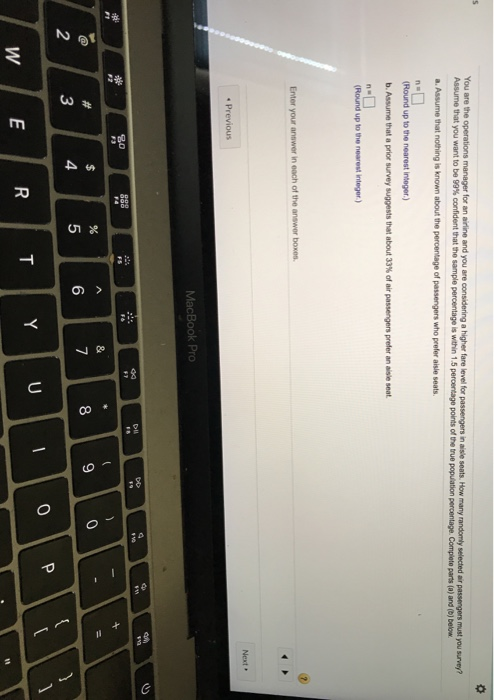You are the operations manager for an airline and you are considering a higher fare level for passengers in aisle seats. How many randomly selected air passengers must you survey? Assume that you want to be 99% confident that the sample percentage is within 1.5 percentage points of the true population percentage. Complete parts (a) and (b) below. a. Assume that nothing is known about the percentage of passengers who prefer aisle seats. (Round up to the nearest Integer.) b. Assume that a prior survey suggests that about 33% of air passengers prefer an aisle seat. (Round up to the nearest Integer) Enter your antiwar in each of the answer boxes. • Previous MacBook Pro W/ RITY UT0 Pili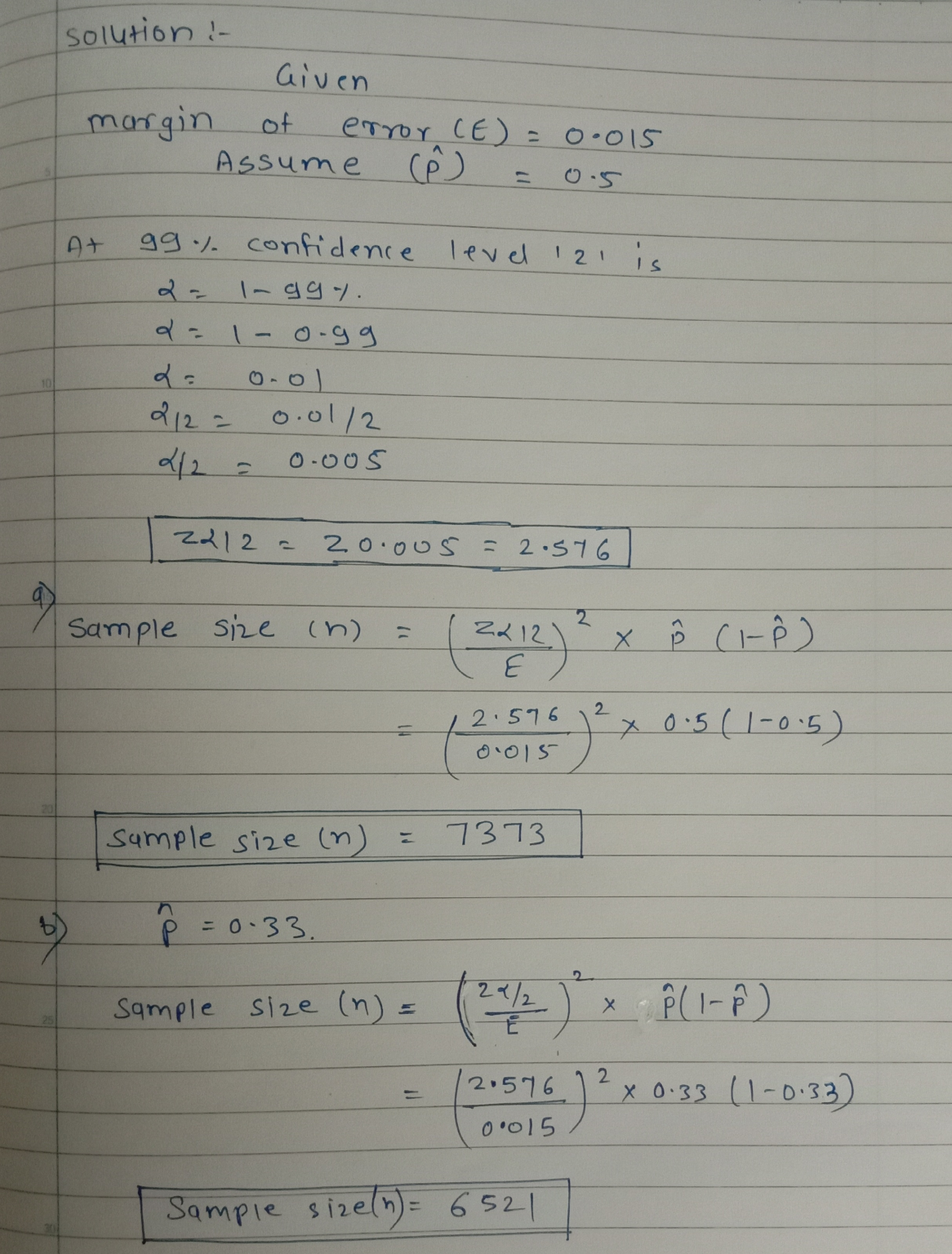##### Add Answer to: You are the operations manager for an airline and you are considering a higher fare level...
Similar Homework Help Questions
• ### You are the operations manager for an airline and you are considering a higher fare level...

You are the operations manager for an airline and you are considering a higher fare level for passengers in aisle seats. How many randomly selected air passengers must you​ survey? Assume that you want to be 99​% confident that the sample percentage is within 3.5 percentage points of the true population percentage. Complete parts​ (a) and​ (b) below. a. Assume that nothing is known about the percentage of passengers who prefer aisle seats. n= ​(Round up to the nearest​ integer.)...

• ### You are the operations manager for an airline and you are considering a higher fare level...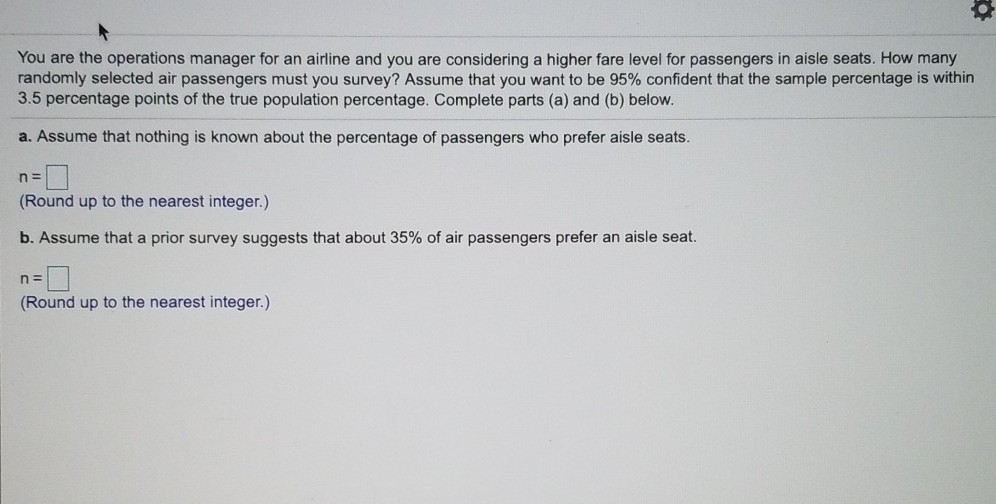You are the operations manager for an airline and you are considering a higher fare level for passengers in aisle seats. How many randomly selected air passengers must you survey? Assume that you want to be 95% confident that the sample percentage is within 3.5 percentage points of the true population percentage. Complete parts (a) and (b) below. a. Assume that nothing is known about the percentage of passengers who prefer aisle seats. n= (Round up to the nearest integer.)...

• ### You are the operations manager for an airline and you are considering a higher fare level...

You are the operations manager for an airline and you are considering a higher fare level for passengers in aisle seats. How many randomly selected air passengers must you​ survey? Assume that you want to be 90​% confident that the sample percentage is within 1.5 percentage points of the true population percentage. Complete parts​ (a) and​ (b) below. a. Assume that nothing is known about the percentage of passengers who prefer aisle seats. n=3007 ​(Round up to the nearest​ integer.)...

• ### You are the operations manager for an airline and you are considering a higher fare level...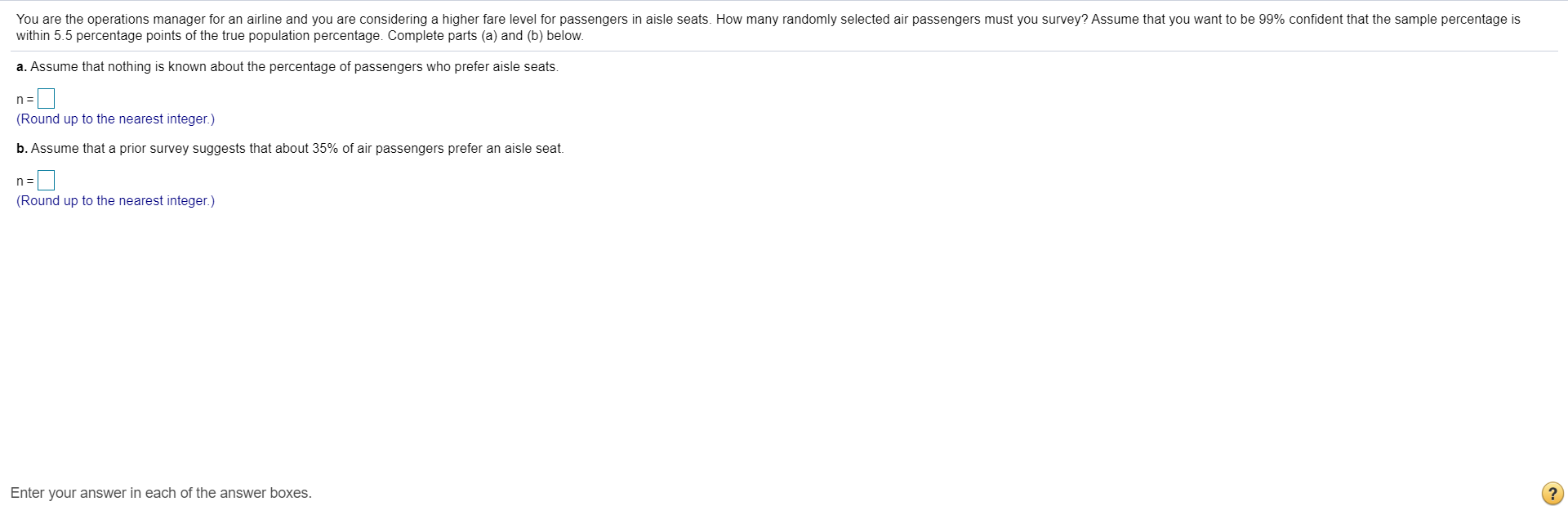You are the operations manager for an airline and you are considering a higher fare level for passengers in aisle seats. How many randomly selected air passengers must you survey? Assume that you want to be 99% confident that the sample percentage is within 5.5 percentage points of the true population percentage. Complete parts (a) and (b) below. a. Assume that nothing is known about the percentage of passengers who prefer aisle seats. n = (Round up to the nearest...

• ### You are the operations manager for an airline and you are considering a higher fare level...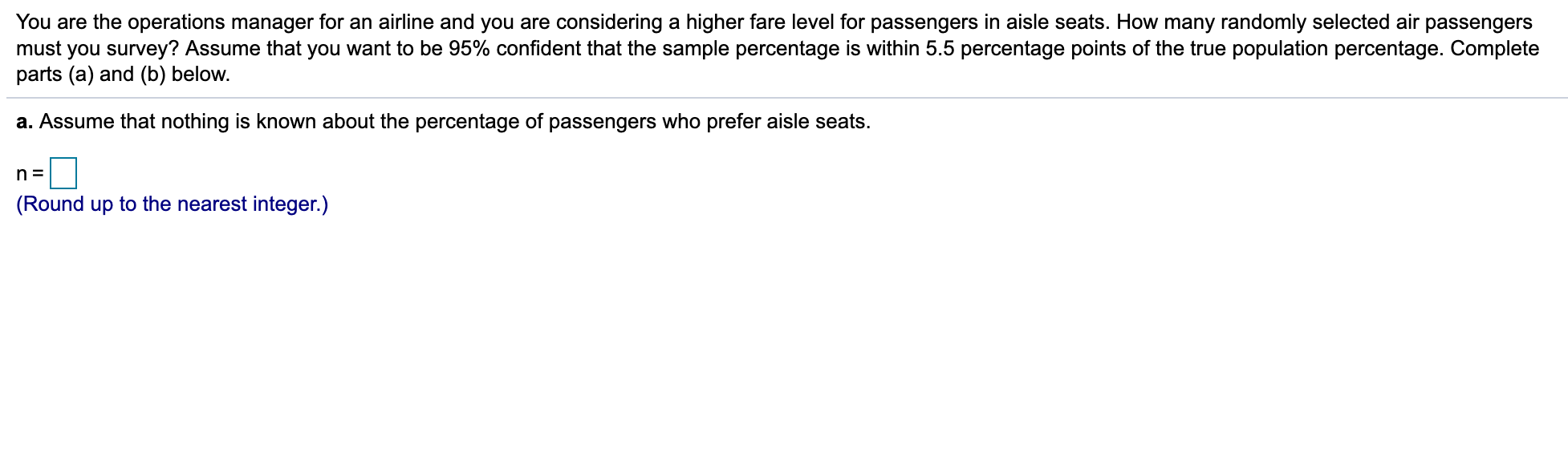You are the operations manager for an airline and you are considering a higher fare level for passengers in aisle seats. How many randomly selected air passengers must you survey? Assume that you want to be 95% confident that the sample percentage is within 5.5 percentage points of the true population percentage. Complete parts (a) and (b) below. a. Assume that nothing is known about the percentage of passengers who prefer aisle seats. n = (Round up to the nearest...

• ### You are the operations manager for an airline and you are considering a higher fare level...

You are the operations manager for an airline and you are considering a higher fare level for passengers in aisle seats. How many randomly selected air passengers must you​ survey ? Assume that a prior survey suggests that about 73​% of air passengers prefer an aisle seat. Assume that you want to be 97% confident that the sample percentage is within 2.5 percentage points of the true population percentage.

• ### 7.1.35-T You are the operations manager for an airline and you are considering a higher fare...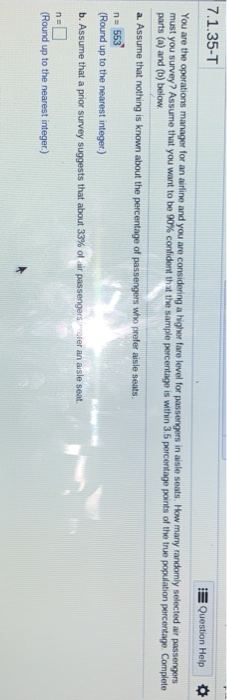7.1.35-T You are the operations manager for an airline and you are considering a higher fare level for passengers in aisle seats. How many parts (a) and (b) below Question Help * confident that the sample percentage is within 3.5 percentage points of the true population percentage Complete n 553 (Round up to the nearest integer.) b. Assume that a pnor survey suggests that about 33% of ar passengers rier an asle seat. n- Round up to the nearest integer.)

• ### Course Home Announcements You are the operations manager for an airline and you are considering a...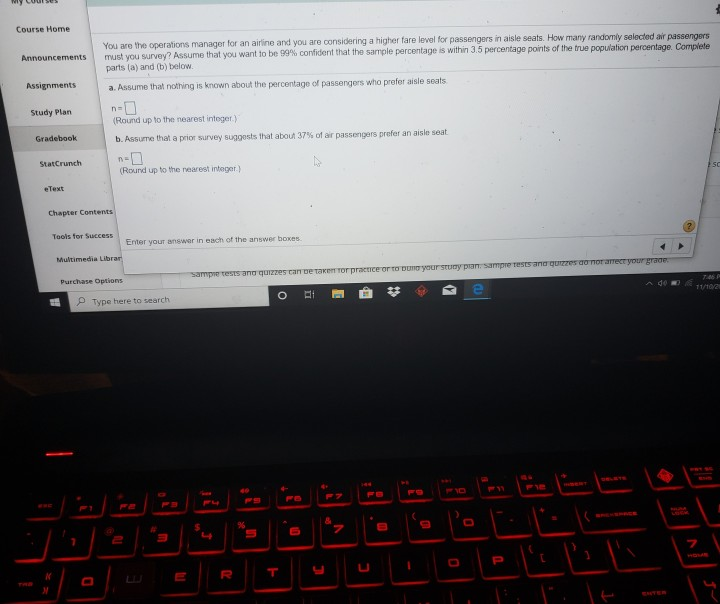Course Home Announcements You are the operations manager for an airline and you are considering a higher fare level for passengers in aisle seats. How many randomly selected air passengers must you survey? Assume that you want to be confident that the sample percentage is within 3.5 percentage points of the true population percentage. Complete parts la) and (b) below Assignments a. Assume that nothing is known about the percentage of passengers who prefer aisle seats Study Plan Gradebook n=1...

• ### 7.1.35-T Question Help You are the operations manager for an airline and you are considering a...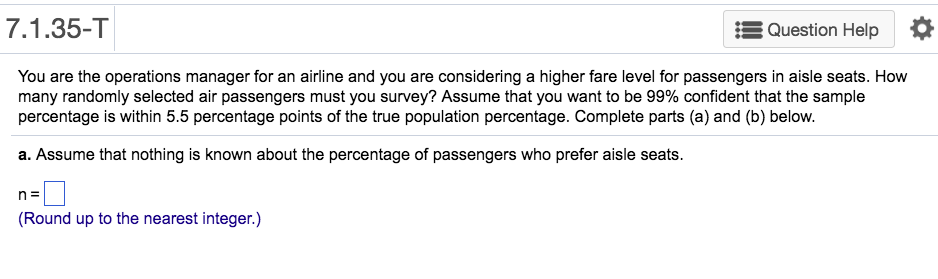7.1.35-T Question Help You are the operations manager for an airline and you are considering a higher fare level for passengers in aisle seats. How many randomly selected air passengers must you survey? Assume that you want to be 99% confident that the sample percentage is within 5.5 percentage points of the true population percentage. Complete parts (a) and (b) below. a. Assume that nothing is known about the percentage of passengers who prefer aisle seats. n: Round up to...

• ### Help please Homework: HW16 - Estimating a Population Proportion Score: 0 of 2 pts 7.1.35-T Save...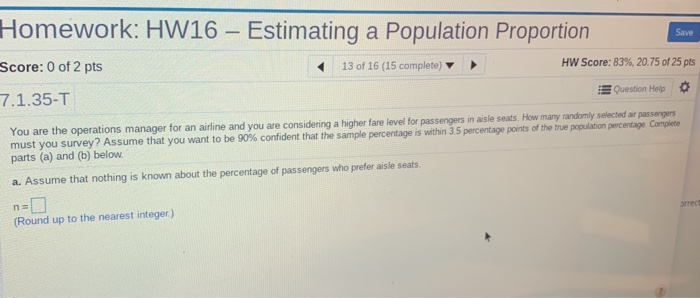Help please Homework: HW16 - Estimating a Population Proportion Score: 0 of 2 pts 7.1.35-T Save 13 of 16 (15 complete) HW Score: 83%, 20.75 of 25 pts Question Hep You are the operations manager for an airline and you are considering a higher fare level for passengers in aisle seats. How many randomly selected air passengers must you survey? Assume that you want to be 90% confident that the sample percentage is within 3 serena w pots of ho...

Free Homework App